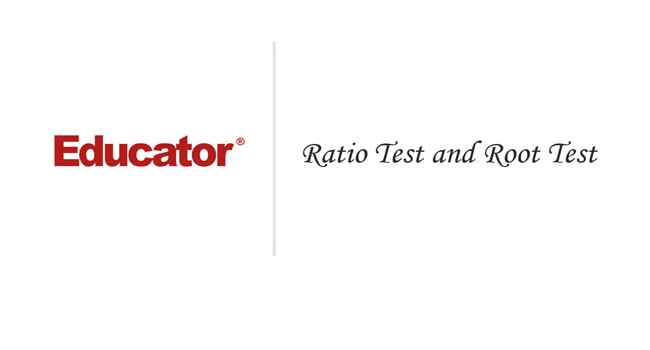Professor Murray

Ratio Test and Root Test

Slide Duration:

Integration by Parts

24m 52s

Intro
0:00
Important Equation
0:07
Where It Comes From (Product Rule)
0:20
Why Use It?
0:35
Lecture Example 1
1:24
Lecture Example 2
3:30
Shortcut: Tabular Integration
7:34
Example
7:52
Lecture Example 3
10:00
Mnemonic: LIATE
14:44
Ln, Inverse, Algebra, Trigonometry, e
15:38
-1
-2
Integration of Trigonometric Functions

25m 30s

Intro
0:00
Important Equation
0:07
Powers (Odd and Even)
0:19
What To Do
1:03
Lecture Example 1
1:37
Lecture Example 2
3:12
Half-Angle Formulas
6:16
Both Powers Even
6:31
Lecture Example 3
7:06
Lecture Example 4
10:59
-1
-2
Trigonometric Substitutions

30m 9s

Intro
0:00
Important Equations
0:06
How They Work
0:35
Example
1:45
Remember: du and dx
2:50
Lecture Example 1
3:43
Lecture Example 2
10:01
Lecture Example 3
12:04
-1
-2
Partial Fractions

41m 22s

Intro
0:00
Overview
0:07
Why Use It?
0:18
Lecture Example 1
1:21
Lecture Example 2
6:52
Lecture Example 3
13:28
-1
-2
Integration Tables

20m

Intro
0:00
Using Tables
0:09
Match Exactly
0:32
Lecture Example 1
1:16
Lecture Example 2
5:28
Lecture Example 3
8:51
-1
-2
Trapezoidal Rule, Midpoint Rule, Left/Right Endpoint Rule

22m 36s

Intro
0:00
Trapezoidal Rule
0:13
Graphical Representation
0:20
How They Work
1:08
Formula
1:47
Why a Trapezoid?
2:53
Lecture Example 1
5:10
Midpoint Rule
8:23
Why Midpoints?
8:56
Formula
9:37
Lecture Example 2
11:22
Left/Right Endpoint Rule
13:54
Left Endpoint
14:08
Right Endpoint
14:39
Lecture Example 3
15:32
-1
-2
Simpson's Rule

21m 8s

Intro
0:00
Important Equation
0:03
Estimating Area
0:28
Difference from Previous Methods
0:50
General Principle
1:09
Lecture Example 1
3:49
Lecture Example 2
6:32
Lecture Example 3
9:07
-1
-2
Improper Integration

44m 18s

Intro
0:00
Horizontal and Vertical Asymptotes
0:04
Example: Horizontal
0:16
Formal Notation
0:37
Example: Vertical
1:58
Formal Notation
2:29
Lecture Example 1
5:01
Lecture Example 2
7:41
Lecture Example 3
11:32
Lecture Example 4
15:49
Formulas to Remember
18:26
Improper Integrals
18:36
Lecture Example 5
21:34
Lecture Example 6 (Hidden Discontinuities)
26:51
-1
-2
Section 2: Applications of Integrals, part 2
Arclength

23m 20s

Intro
0:00
Important Equation
0:04
Why It Works
0:49
Common Mistake
1:21
Lecture Example 1
2:14
Lecture Example 2
6:26
Lecture Example 3
10:49
-1
-2
Surface Area of Revolution

28m 53s

Intro
0:00
Important Equation
0:05
Surface Area
0:38
Relation to Arclength
1:11
Lecture Example 1
1:46
Lecture Example 2
4:29
Lecture Example 3
9:34
-1
-2
Hydrostatic Pressure

24m 37s

Intro
0:00
Important Equation
0:09
Main Idea
0:12
Different Forces
0:45
Weight Density Constant
1:10
Variables (Depth and Width)
2:21
Lecture Example 1
3:28
-1
-2
Center of Mass

25m 39s

Intro
0:00
Important Equation
0:07
Main Idea
0:25
Centroid
1:00
Area
1:28
Lecture Example 1
1:44
Lecture Example 2
6:13
Lecture Example 3
10:04
-1
-2
Section 3: Parametric Functions
Parametric Curves

22m 26s

Intro
0:00
Important Equations
0:05
Slope of Tangent Line
0:30
Arc length
1:03
Lecture Example 1
1:40
Lecture Example 2
4:23
Lecture Example 3
8:38
-1
-2
Polar Coordinates

30m 59s

Intro
0:00
Important Equations
0:05
Polar Coordinates in Calculus
0:42
Area
0:58
Arc length
1:41
Lecture Example 1
2:14
Lecture Example 2
4:12
Lecture Example 3
10:06
-1
-2
Section 4: Sequences and Series
Sequences

31m 13s

Intro
0:00
Definition and Theorem
0:05
Monotonically Increasing
0:25
Monotonically Decreasing
0:40
Monotonic
0:48
Bounded
1:00
Theorem
1:11
Lecture Example 1
1:31
Lecture Example 2
11:06
Lecture Example 3
14:03
-1
-2
Series

31m 46s

Intro
0:00
Important Definitions
0:05
Sigma Notation
0:13
Sequence of Partial Sums
0:30
Converging to a Limit
1:49
Diverging to Infinite
2:20
Geometric Series
2:40
Common Ratio
2:47
Sum of a Geometric Series
3:09
Test for Divergence
5:11
Not for Convergence
6:06
Lecture Example 1
8:32
Lecture Example 2
10:25
Lecture Example 3
16:26
-1
-2
Integral Test

23m 26s

Intro
0:00
Important Theorem and Definition
0:05
Three Conditions
0:25
Converging and Diverging
0:51
P-Series
1:11
Lecture Example 1
2:19
Lecture Example 2
5:08
Lecture Example 3
6:38
-1
-2
Comparison Test

22m 44s

Intro
0:00
Important Tests
0:01
Comparison Test
0:22
Limit Comparison Test
1:05
Lecture Example 1
1:44
Lecture Example 2
3:52
Lecture Example 3
6:01
Lecture Example 4
10:04
-1
-2
Alternating Series

25m 26s

Intro
0:00
Main Theorems
0:05
Alternation Series Test (Leibniz)
0:11
How It Works
0:26
Two Conditions
0:46
Never Use for Divergence
1:12
Estimates of Sums
1:50
Lecture Example 1
3:19
Lecture Example 2
4:46
Lecture Example 3
6:28
-1
-2
Ratio Test and Root Test

33m 27s

Intro
0:00
Theorems and Definitions
0:06
Two Common Questions
0:17
Absolutely Convergent
0:45
Conditionally Convergent
1:18
Divergent
1:51
Missing Case
2:02
Ratio Test
3:07
Root Test
4:45
Lecture Example 1
5:46
Lecture Example 2
9:23
Lecture Example 3
13:13
-1
-2
Power Series

38m 36s

Intro
0:00
Main Definitions and Pattern
0:07
What Is The Point
0:22
0:45
Interval of Convergence
2:42
Lecture Example 1
3:24
Lecture Example 2
10:55
Lecture Example 3
14:44
-1
-2
Section 5: Taylor and Maclaurin Series
Taylor Series and Maclaurin Series

30m 18s

Intro
0:00
Taylor and Maclaurin Series
0:08
Taylor Series
0:12
Maclaurin Series
0:59
Taylor Polynomial
1:20
Lecture Example 1
2:35
Lecture Example 2
6:51
Lecture Example 3
11:38
Lecture Example 4
17:29
-1
-2
Taylor Polynomial Applications

50m 50s

Intro
0:00
Main Formulas
0:06
Alternating Series Error Bound
0:28
Taylor's Remainder Theorem
1:18
Lecture Example 1
3:09
Lecture Example 2
9:08
Lecture Example 3
17:35
-1
-2
Bookmark & Share Embed

## Copy & Paste this embed code into your website’s HTML

Please ensure that your website editor is in text mode when you paste the code.
(In Wordpress, the mode button is on the top right corner.)
×
• - Allow users to view the embedded video in full-size.
Since this lesson is not free, only the preview will appear on your website.

• ## Related Books1 answer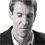Last reply by: Dr. William MurrayThu Aug 4, 2016 6:03 PMPost by Peter Ke on July 30, 2016For example 1, how does e^n+1/e^n = e and how is (n+1)! the same as n!(n+1). I know you explained it in the video but I still don't understand.Please explain!1 answerLast reply by: Dr. William MurrayThu Apr 4, 2013 5:45 PMPost by Rohail Tariq on April 4, 2013that was a brilliant video. i understood everything :)1 answerLast reply by: Dr. William MurrayThu Apr 4, 2013 5:45 PMPost by Ahmad Al-kheat on April 9, 2012so just to recap here :)

### Ratio Test and Root Test

Main definitions and theorems:

Definitions: Let an be a series.

• anis Absolutely Convergent means that an converges and ∑|an| converges.

• anis Conditionally Convergent means that an converges but ∑|an| diverges.

• anis Divergent means that an diverges and ∑|an| diverges.

Theorem: If ∑|an| converges, then an converges.

Ratio Test: Suppose an is a series. Calculate lim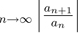and call it L.

• If L < 1, then the series converges absolutely.

• If L > 1, then the series diverges.

• If L = 1, then the Ratio Test doesn’t tell you. The series could converge absolutely, converge conditionally, or diverge.

Root Test: Suppose an is a series. Calculate lim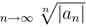and call it L.

• If L < 1, then the series converges absolutely.

• If L > 1, then the series diverges.

• If L = 1, then the Root Test doesn’t tell you. The series could converge absolutely, converge conditionally, or diverge.

Hints and tips:

• Think of an Absolutely Convergent series as being “super-convergent”. It is so strongly convergent that it still converges even if we make everything positive.

• Think of a Conditionally Convergent series as being “just barely convergent”. It only converges because some of the terms are negative.

• Here are some flags that might suggest the Ratio Test:

• (−1)n , since many other tests require positive terms.

• n!, (2n)!, since factorials cancel nicely in ratios. (Remember that (n + 1)! = (n + 1)n! and [2(n + 1)]! = (2n)!(2n + 1)(2n + 2).)

• constantn (including en )

• Any kind of power series/Taylor Series (coming later)

• Here are some flags that might suggest that you not use the Ratio Test:

• n, n² (or any polynomial), n, ln n. All of these have lim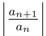= 1, so the Ratio Test fails. But if you have some of this clutter mixed with some good stuff, Ratio is great for clearing the clutter away and leaving you with something good.

• nn , or more generally (a function of n)(another function of n) (more appropriate for Root Test)

(Note that the Ratio Test is based on comparison with geometric series. So things that look geometric, e.g. constantn , make it work. p-series do not.)

• Remember that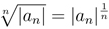.

• Here are some flags that might suggest the Root Test:

• (−1)n , since many other tests require positive terms.

• (a function of n)(another function of n), e.g. nn , (n − 2)²n , etc. Not (constant)n (use geometric series or Ratio Test) or nconstant (use p-series or LCT).

• Here are some flags that might suggest that you not use the Root Test:

• n!, (2n)!, etc. (Use Ratio Test.)

• n² , n, √n. All of these have lim |an|1/n = 1. But if you have some of this mixed with some stuff that looks good for Root Test, then you can use Root.

• Remember that you can write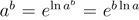. Then you can often sort out the exponent using L’Hôpital.

• It is probably worth memorizing that lim= e, or even that
lim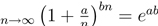.

• Also remember that e ≈ 2.7, so ek > 1 if k is positive and ek < 1 if k is negative.

### Ratio Test and Root Test

Does the series ∑3n converges or diverges
• Apply the Ratio Test, find an + 1
• an + 1 = 3n + 1
L = limn → ∞ [(3n + 1)/(3n)] = 3L > 1, thus ∑3n diverges
Does the series ∑[1/(2n)] converges or diverges
• Apply the Ratio Test, find an + 1
• an + 1 = [1/(2n + 1)]
• Find and analyze L
= limn → ∞ [(2n)/(2n + 1)] = [1/2]L < 1, thus ∑[1/(2n)] converges
Does the series ∑[n/(3n)] converges or diverges
• Apply the Ratio Test, find an + 1
• an + 1 = [(n + 1)/(3n + 1)]
• Find and analyze L
• L = limn → ∞ [([(n + 1)/(3n + 1)])/([n/(3n)])]
• = limn → ∞ [(n + 1)/(3n(3))]( [(3n)/n] )
• = limn → ∞ [(n + 1)/3n]
• = [n/3n]
• = [1/3]
L < 1, thus ∑[1/(2n)] converges
Does the series ∑[(5n)/(n2)] converges or diverges
• Apply the Ratio Test, find an + 1
• an + 1 = [(5n + 1)/((n + 1)2)]
• = [(5n + 1)/(n2 + 2n + 1)]
• Find and analyze L
• L = limn → ∞ [([(5n + 1)/(n2 + 2n + 1)])/([(5n)/(n2)])]
• = limn → ∞ [(5n + 1)/(n2 + 2n + 1)]( [(n2)/(5n)] )
= [(5n2)/(n2)] = 5L > 1, thus the series diverges
Can the Ratio Test be applied to the series ∑[(n + 1)/(n − 1)]?
• Apply the Ratio Test, find an + 1
• an + 1 = [(n + 2)/n]
• Find and analyze L
• L = limn → ∞ [([(n + 2)/n])/([(n + 1)/(n − 1)])]
• = limn → ∞ [(n + 2)/n]( [(n − 1)/(n + 1)] )
= [(n2)/(n2)] = 1L = 1, thus another test should be applied.
Does the series ∑[2/((n + 1)!)] converges or diverges
• Apply the Ratio Test, find an + 1
• an + 1 = [2/((n + 2)!)]
• Find and analyze L
• L = limn → ∞ [([2/((n + 2)!)])/([2/((n + 1)!)])]
• = limn → ∞ [2/((n + 2)!)]( [((n + 1)!)/2] )
• = limn → ∞ [1/(n + 2)]
• = 0
L < 1, thus the series converges
Does the series ∑[(2n)/n!] converges or diverges
• Apply the Ratio Test, find an + 1
• an + 1 = [(2n + 1)/((n + 1)!)]
• Find and analyze L
• L = limn → ∞ [([(2n + 1)/((n + 1)!)])/([(2n)/n!])]
= limn → ∞ [2/(n + 1)] = 0L < 1, thus the series converges
Does the series ∑[n!/((n − 10)!)] converges or diverges
• Apply the Ratio Test, find an + 1
• an + 1 = [((n + 1)!)/((n − 9)!)]
• Find and analyze L
• L = limn → ∞ [([((n + 1)!)/((n − 9)!)])/([n!/((n − 10)!)])]
• = limn → ∞ [((n + 1)!)/((n − 9)!)]( [((n − 10)!)/n!] )
• = limn → ∞ ( n + 1 )(n − 10)
• = ∞
L > 1, thus the series diverges
Does the series ∑[(4n)/(6n)] converges or diverges
• Apply the Ratio Test, find an + 1
• an + 1 = [(4n + 1)/(6n + 1)]
• Find and analyze L
• L = limn → ∞ [([(4n + 1)/(6n + 1)])/([(4n)/(6n)])]
= limn → ∞ [4/6] = [4/6]L < 1, thus the series converges
Does the series ∑[(2n + 1)/(3n − 1)] converges or diverges
• Apply the Ratio Test, find an + 1
• an + 1 = [(2n + 2)/(3n)]
• Find and analyze L
• L = limn → ∞ [([(2n + 2)/(3n)])/([(2n + 1)/(3n − 1)])]
• = limn → ∞ [(2n + 2)/(3n)]( [(3n − 1)/(2n + 1)] )
• = [2/3]
L < 1, thus the series converges

*These practice questions are only helpful when you work on them offline on a piece of paper and then use the solution steps function to check your answer.

### Ratio Test and Root Test

Lecture Slides are screen-captured images of important points in the lecture. Students can download and print out these lecture slide images to do practice problems as well as take notes while watching the lecture.

• Intro 0:00
• Theorems and Definitions 0:06
• Two Common Questions
• Absolutely Convergent
• Conditionally Convergent
• Divergent
• Missing Case
• Ratio Test 3:07
• Root Test 4:45
• Lecture Example 1 5:46
• Lecture Example 2 9:23
• Lecture Example 3 13:13

### Transcription: Ratio Test and Root Test

Hi we are here trying more examples of the ratio test and the root test.0000

We have a kind of complicated one, -100n n4/n! × ln(n).0004

That one looks a little nasty but you will see that the ratio test handles it pretty well.0014

So, ratio test says you look at an+1/an.0022

I am going to work out this whole expression, but substituting an n+1 for n.0028

We get -100n+1 (n+1)4/n+1! × ln(n+1).0036

All of that divided by the same thing just with n, so -100n n4/n! × ln(n).0060

Again, I am going to flip the denominator and bring it up and multiply it into the numerator,0070

I am going to go ahead and pair the factors off with factors that look like them.0078

We get 100n+1/100n.0084

By the way, I am getting rid of the negative signs because we are taking absolute values there.0089

n+1/4/n4.0096

Let us see, this n! is going to flip up into the numerator,0103

So we have n!/n+1!.0109

Then the ln(n) is going to flip up into the numerator.0112

ln(n) × ln(n+1).0117

Then let us look at each one of those factors separately.0122

100n+1/100n is 100.0126

Now (n+1)4 is going to be some big complicated polynomial.0130

The key thing here is that n4 plus some smaller terms.0136

We are dividing it by n4,0145

So both in top and bottom there, the biggest term is n4.0148

We can divide top and bottom by n4,0154

So, that will make the n4 terms go to 1 when you take the limit.0161

All of the smaller terms go to 0.0168

This just goes to 1 as n goes to infinity.0171

Remember, that was the pattern we saw before with polynomials.0177

When you take the ratio of n+1 and n in a polynomial, you always get 1.0180

That is very useful for the ratio test.0186

n+1!, remember we can write that as n!.0189

× n+1, so the n! cancels with the n! in the numerator.0195

We get 1/n+1.0201

Then ln(n)/ln(n+1).0204

For that we could say that that goes to infinity/infinity.0209

We could use l'hopital's rule.0215

L'hopital's rule says you take the derivative of the top and bottom,0219

That is 1/n divided 1/n+1.0220

So that is n+1/n.0226

The limit of that as n goes to infinity is just 1.0231

You put all of these things together and you get, 100/n+1.0240

Now, you can take the limit as n goes to infinity.0249

Now 100 is constant and n is getting bigger and bigger, so this limit is 0.0255

We can say l=0, so the series converges absolutely.0259

By the ratio test.0280

The key thing about the 0 there is that it is less than 1.0288

That was the cut off to check for the ratio test.0295

There is a lot of things we can learn from this example.0300

If you look at the different terms here, which ones work well for the ratio test?0305

Well, the -100, if we follow the -100,0312

If we kind of trace those terms through the ratio test,0317

That gave us a 100, which was very very good for the ratio test.0321

I like using that for the ratio test.0328

Good in the sense that it did contribute to the answer.0330

n4, if you look at n4 and follow that through,0335

That just gave us 1 at the end.0340

Remember, in the ratio test, if you get 1 as an answer, it does not tell you whether or not the series converges.0343

So n4 was not useful for the ratio test.0350

n!, we have got n+1! and n!, that cancelled away nicely, we got 1/n+1,0355

So the n! was good for the ratio test.0370

ln(n), if you trace those through, ln(n), just ended up giving us 1.0374

Remember, if you get 1 as the answer to the ratio test, it gives you no information.0383

If you have just things like polynomials like this n4, or natural logs,0388

Your answer for the ratio test is going to be 1 which gives you no information and it means you did a lot of work for nothing.0396

On the other hand, if you have geometric terms, like -100n,0405

Or factorial terms, like n!,0410

Then the ratio test is very useful in giving an answer.0415

Let me summarize that there.0418

If you have geometric terms, like 100n, or factorial terms,0421

You definitely want to use the ratio test.0434

Because it will give you a useful answer.0439

On the other hand, if you have polynomial terms,0444

Or natural log terms, remember polynomials like n4,0450

When you run those through the ratio test, what you get for your answer is 1.0456

The ratio test gives you no information if your answer comes out to be 1.0463

If you see those turns, do not use the ratio test.0470

You will be wasting your time and you will not get an answer.0473

Do not use the ratio test on those terms.0480

Unless you also have some of the good terms, the geometric and factorial stuff.0483

Good factors.0502

To summarize that, the ratio test works very well on geometric and factorial stuff.0506

It works badly on polynomial and natural log stuff.0512

However, if you have a mix of both,0516

You do want to use the ratio test.0520

Because the ratio test will sort of sweep away the polynomial and the natural log stuff.0523

It will leave you with the good stuff, the geometric stuff and the factorial stuff that will actually give you a meaningful answer.0530

That is kind of when to use the ratio test and when not to use the ratio test.0541

How you can tell ahead of time whether the ratio test is going to be a useful one,0546

Or whether it is going to be a big waste of time.0550

We are going to try one more example here.0000

The sum of 1-2/nn2.0004

What I notice right away about this one is that I have some stuff involving n, the 1-2/n,0010

Raised to some stuff involving n in the exponent.0016

I have n both in the base and the exponent.0022

That is not something I want to use the ratio test.0027

Do not use the ratio test for something like that.0028

Instead, you want to use the root test for something like that.0032

When you have something involving n raised to something involving n in the exponent,0039

You want to use the root test on that.0044

Let us try this out using the root test.0047

Remember that says you look at the absolute value of an1/n,0050

That is the nth root of the terms.0055

This is 1-2/n raised to the n2, all raised to the 1/n power.0059

I am going to get rid of the absolute values right away because 1-2/n,0074

After n gets big enough, that is going to be a positive number.0080

This is 1-2/n.0085

Now n2 raised to the 1/n,0090

Remember you multiply those exponents.0092

That is n2/n, that is just n.0097

Now this is a tricky expression, 1-2/nn.0103

We have seen an example like this before in another lecture.0108

The trick here is when you have something complicated raised to a complicated exponent,0112

You try to separate them out.0117

You write this as eln(ab), the point of doing that is you can pull the exponent b out of the natural log.0121

You can write it as eb, ln(a).0132

Here the a is the 1-2/n and the b is n.0138

We can write this as en × ln(1-2/n).0142

Now we are just going to focus on the exponent.0155

Because that will take a little work to sort out.0158

We will come back later and incorporate the e term.0162

Let us just look at that exponent.0165

n × ln(1-2/n).0166

n × ln(1-2/n), well n goes to infinity.0175

ln(1-2/n), well 2/n goes to 0.0180

That is ln(1) which is 0.0186

We have an infinity × 0 situation.0189

You cannot do anything with infinity × 0, it could come out to be anything.0193

What I mean is you cannot do anything directly.0197

What you can do is write it as ln(1-2/n)/1/n.0200

That is going to, the numerator is still 0, 1/n is now 0, that is going to 0 over 0.0214

That is a situation where you can use l'Hopital's rule.0223

We are going to invoke l'Hopital's rule here.0228

Remember l'Hopital's rule, you take the derivative of the top and bottom separately.0233

It is not like the quotient rule from Calculus 1.0241

It is kind of different.0243

You take the derivative of the top and bottom separately.0244

I will take the derivative of the bottom first because it is easier.0248

Derivative of 1/n is -1/n2,0250

Now the derivative of the top, natural log of something,0256

Is 1/that something, 1-2/n.0261

× derivative of the inside part.0263

That is the derivative of 1-2/n is - 2/n2, and the negative of that turns that into a positive.0267

The derivative of 2/n is -2/n2, derivative of -2/n is positive 2/n2.0283

Let us clean this up a little.0294

The 1/n2 cancels with that 1/n2 and we are left with negative from the denominator 2/1-2/n.0296

Now, as n goes to infinity, the 2/n goes to 0.0307

This whole thing goes to -2.0317

Remember, this was the exponent.0320

If we incorporate back in this e that we had before,0324

The true limit here, an to the 1/n goes to e-2.0329

So, our limit l that we checked for the root test is e-2.0340

That is the same as 1/e2.0347

We have to figure out approximately how big that is.0350

e is approximately 2.7.0354

1/e2, I do not know exactly what it is, but I know that it is definitely less than 1.0357

That is the cut off for the root test, and so,0365

If l < 1, that tells us that the series aN is absolutely convergent by the root test.0370

Let us recap what we did here.0402

The first thing we did when we looked at this series was to say,0407

I see an n in the base and an n in the exponent.0410

That is something complicated, not something that the ratio test is going to work well on.0414

That tells me right away, try for the root test.0421

I set up aN to the 1/n.0425

That simplifies down a little bit and we get to this step right here.0428

When we look at that step, again looks like something a little complicated.0433

I can sort it out using this eln rule.0439

You sort it out using this eln rule,0442

And if you look at the exponent, you get infinity × 0.0447

You can rewrite that as 0/0.0451

Which allows you to invoke l'Hopital's rule.0454

You work l'Hopital's rule through and you get -2.0459

But that -2 is actually just the exponent because you still have this e from earlier.0462

So you get e-2 as our final limit.0470

The important thing about that was that it was less than 1,0472

And, the root test says that if your limit is less than 1, then the whole series is absolutely convergent.0477

This is educator.com, thanks for watching.0486

Hi, this is educator.com and we are here to learn about the ratio test and the root test.0000

Now, there are some definitions we need before we get started.0007

From now on, before you are given a series,0010

You are going to be asked two different questions packed into one.0014

The first is whether the original series converges.0017

The second question is if you take the absolute value of each term of the series, does it still converge?0021

The point there is that you are making all of the terms positive,0031

That potentially makes the series bigger and might make it diverge.0033

There are three classifications that we are going to be using for series from now on.0039

We are going to say it is absolutely convergent if the original series converges and the absolute series converges.0047

The words absolutely convergent are supposed to make you think of something that is very strongly convergent.0054

In the sense that, I say super-convergent, even if you make all of the terms positive,0064

Even if you make all of the terms as big as you can, that series still converges.0070

That is absolute convergence.0075

Conditional convergence means that the series converges,0078

But the absolute series diverges.0084

The way you want to think about that is the series just barely converges.0090

It does converge, but if you make all of the terms positive,0092

Then it gets so big that it diverges.0096

It only just barely converged because some of the original terms were negative.0100

It had to have some of the negative terms to make it converge.0105

If you make all of the terms positive, it diverged.0108

Then finally, we will talk about divergent series,0110

Means that the series diverges and the absolute series also diverges.0115

The case that is missing is what if an diverges,0127

But the absolute series converges.0137

The answer is that that possibility cannot happen.0148

Essentially, you want to think about it as the absolute series is always at least as big as the original series.0150

If the absolute series converges, then the original series must converge as well.0158

That is what this theorem is saying.0164

If the absolute series converges, then the original series must converge as well.0166

You cannot have the situation where the original series diverges but the absolute series converges.0172

That never happens.0178

There are really only three possible answers for all the series that we are going to study today.0180

You will be given a series,0190

And, one nice thing here is that you do not have to check anything about whether the series has positive terms or anything like that.0191

This is a very strong test.0200

It works for any kind of series.0203

For any kind of series where you can calculate this limit.0206

What you do is you look at the n+1 term, and you look at the n term,0208

You divide them together, you take the absolute value, and then you take the limit of that.0213

Whatever you get, you call it l.0218

Now if l is less than 1, then the series converges absolutely.0221

If l is bigger than 1, the series diverges.0226

The natural inclination at this point is to say that if l=1 then the series converges conditionally, because that is the remaining case.0230

That is not true.0238

If l=1, then it kind of breaks down.0240

The ratio test gives you no answer, and there are examples where the series converges absolutely.0244

There are other examples where the series converges conditionally.0251

There are others where it diverges.0254

Essentially if l=1, then you have wasted your time using the ratio test,0256

Because it does not give you an answer, you have to find something else.0262

That is the downside of the ratio test, if you get l=1.0266

However, if you get l = bigger than 1 or less than 1, that includes infinity and 0,0268

Those are OK to use here, then you do get a good answer.0272

You can say that the series converges absolutely, or diverges. That is the ratio test.0278

We will do some examples in a second but I want to introduce you also to the root test.0284

Which looks a lot like the ratio test but instead of calculating a ratio, you calculate a root.0287

You look at absolute value of the terms, and then you take the nth root.0297

Remember, that is the same as taking an to the 1/n power.0302

You take the limit again and you call that number l.0306

Then you have the exact same conclusions as the ratio test.0310

If l < 1, it converges absolutely, if l > 1, it diverges, and if l = 1, then you might hope to say it converges conditionally,0314

But that would be very unjustified.0326

You would have to look at some other tests,0330

Because just based on the root test, we do not know yet whether it converges conditionally, converges absolutely, or diverges.0332

Let us try that out with some examples.0343

You will see how it works.0345

First example I have is -en/n!.0347

So we are going to go for the ratio test.0351

We are going to look at, remember an +1/an , and the absolute value of that.0353

aN+1 means you look at each term of the series and you plug in n+1 wherever you see n.0360

So that is -en+1/n+1!.0369

an just means the original term, -en/n!.0376

Take absolute value of that.0382

What I am going to do is flip these two fractions.0385

Flip the denominator so we can compare it with a numerator.0388

I will write that as en+1/en × n!/n+1!.0393

There are a couple things to explain here.0403

Notice with the n! and the en,0406

I got that just by flipping the denominator up and rearranging them to match their respective factors in the numerator.0410

The other thing that I noticed was you took out the negative signs and that is because of the absolute values.0419

The absolute values make everything positive so I can just throw away the negative signs there.0425

Now, let us work with this a bit.0428

en+1/en is just e.0431

Now n!/n+1!, remember n+1 factorial.0435

That means you multiply all the numbers together, up to n × n+1.0443

You can write that as n! × n+1.0452

We will write that as n! × n+1, and now the n! cancel.0458

So you get, e/n+1.0464

Now, remember the ratio test says you take the limit of this as n goes to infinity.0468

We will take the limit as n goes to infinity.0475

e is just a number, it is 2.7, well 2.7 and on and on and on.0480

n+1 is getting bigger and bigger and bigger,0487

So we are dividing a constant by a bigger and bigger number.0491

That limit is 0. Our l=0.0493

Now the important thing in the ratio test is you check whether that limit is < or > 1.0499

0 is certainly less than 1, so the series is absolutely convergent by the ratio test.0506

That is the conclusion that we can make here.0520

Just to recap here, we look at the series that we are given,0530

We call that an .0535

Ratio test says you divide an +1/an .0540

aN+1 means you plug in n+1 wherever you saw n.0544

You did a little bit of algebra, the absolute values got rid of the negative signs, the factorials cancel each other out,0548

The limit came out to be 0, that is less than 1, and the ratio test then says that it is absolutely convergent.0556

The next example, we have n! over n2 + 2.0564

Let us go for the ratio test again.0568

so an +1/an .0573

Is n+1!/n+12 + 2/n!/2 + 2.0578

I am going to get rid of the absolute values because everything here is positive.0596

Again I am going to flip the denominator up and match it with the numerator.0600

We get n+1! divided by n! and then n2 + 2/n+12 + 2.0604

These are multiplied together.0617

n+1!/n!, remember you can write n+1! as n+1 × n!.0621

We learned that in a previous example so that cancels down to just n+1.0634

Now, n2 + 2/n+12 + 2.0640

This is a very common pattern where you have a polynomial.0645

The n version of it, in one part and the n+1 part in the other part.0649

The same pattern always works in that you have n2 + some other stuff divided by,0656

Now if you work out n2, you can work it out, you are going to get n2 + some smaller terms, guaranteed.0664

If you divide top and bottom by n2, in the limit that thing is going to go to 1.0673

That always happens when you have a polynomial and you plug in n+1 and n.0685

It always goes to 1.0692

In that sense, the ratio test, what it is kind of doing, is sweeping a polynomial term away from our series because it is just sending that limit to 1.0693

What we have is n+1 × 1.0705

If we take the limit as n goes to infinity, of n+1 × 1, that is infinity which is certainly bigger than 1.0710

So l here, our l is infinity.0722

That is bigger than one.0725

The series diverges by the ratio test.0729

Remember in some of our other tests, like the limit comparison test,0740

You were not allowed to have infinite limits or 0 limits.0747

You were only allowed to have finite non-zero limits in the limit comparison test.0752

The ratio test is much more forgiving.0754

You can have any limit as long as the limit exists.0758

It can be 0, it can be any number, it can be infinity,0762

All you have to check is it less than 1 or is it bigger than 1.0768

If it turns out to be 1, that is the 1 case where you out of luck.0772

If it comes out to be one, then the ratio test does not give you an answer.0776

But 0 or infinity, they are ok for limits in the ratio test,0780

And they do give you an answer.0783

In this case, infinity is certainly bigger than 1 so we can say the series diverges by the ratio test.0786

Our third example is an = n+1n/9n.0795

That is one that is not very conducive to the ratio test.0800

The reason is that we have n's in the base and in the exponents.0806

I do not like using that for the ratio test.0811

Instead we are going to try the root test.0814

I am hoping that the root test will clear away some of those n's in the exponent.0818

Remember the root test says you look at an , well the nth root of that,0829

That is the same as an 1/n,0832

So that is n-1n/9n, absolute value of that to the 1/n,0836

The absolute values do not do anything here because the terms are positive.0847

n1/n is just 1, so we get n-11/9.0855

Then we take the limit of that.0863

As n goes to infinity, 9 is just a constant, so that limit is going to infinity.0866

Here, the l is infinity, which is certainly bigger than 1.0873

The series, we can say it diverges, by the root test, because we got a limit bigger than 1.0880

So, again, what made that series something that we wanted to look at using the root test.0900

The answer is that we had something with n in it, raised to the n power.0910

That is almost always a case that you want to refer to the root test.0917

When you see something with n in it, raised to the n power, that is the kind of thing that you want to use the root test for.0925

Because the ratio test is going to be really ugly.0935

Here we had an n-1n, we know that we want to use the root test.0936

The root test says you look at an 1/n, or the nth root of an ,0944

You work out the limit and again if it comes out to be bigger than 1 it diverges,0950

Less than 1 converges absolutely.0955

If you are unfortunate enough that it is equal to one then you have no information and the root test fails you.0958

So we will try some more examples later on.0966

OR

### Start Learning Now

Our free lessons will get you started (Adobe Flash® required).